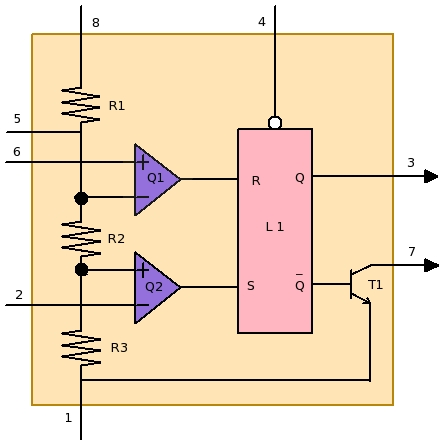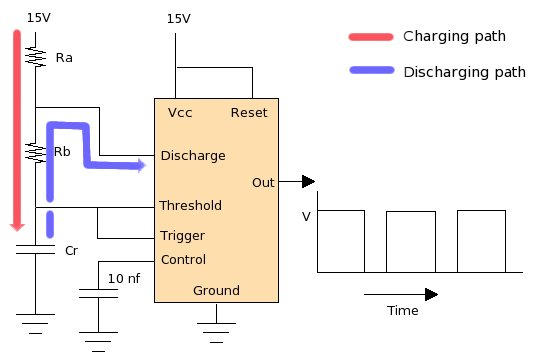black nike shoes roshe cheapnicesports.com

# 555 Timer

People generally go with name, may assume lot from the name itself. Same is true for "IC 555 timer". Name of this chip can make any one feel that the chip is embedded with lot of things and must be quite complex. Lets first clear some of those assumptions which are not true..

1. There is no in-built clock in side "IC 555 timer"
2. There is no crystal present in "IC 555 timer"
3. The chip stand alone won't be able to provide any information about your local time

Now, lets see what "IC 555 timer" is.

1. "IC 555 timer" is used to make waveform generators.
2. With resistor and capacitors, "IC 555" can generate periodic waveforms. Standalone "IC 555" cannot generate any waveform.
3. Various type of waveforms can be generated e.g square, rectangle and ramp etc..
4. Frequency, dutycycle and amplitude of these waveform can be changed by changing capacitor, resistor and input-voltage values.
5. These waveform can be fed to another part of circuit as clock source.

Depending on how throughly one understand its internal logic will enable him to think of other hundred of applications.

Now lets understand its internal logic ..Above diagram is internal representation of "555 timer" chip. This is a 8 pin IC which is used to make wave generator and oscillator. Lets see what's a function of each pin, and together how these can be used to make a square wave generator.

 Pins Pin Names 1 Ground 2 Trigger 3 Output 4 Reset 5 Control 6 Threshold 7 Discharge 8 Vcc
1. [PIN 1] : This is a ground pin, this pin should be tied to ground for proper operation.
2. [PIN 2] : As you can see this pin is connected to "-ve" of "voltage comparator" Q2, and Q2's other pin is connected to a constant voltage 1/3 Vcc (since three resistors are of equal value 5K ohms). Till pin 2 is below 1/3Vcc, output of Q2 will remain high enabling "set" of flipflop. This will result into high voltage value at the output (pin 3).
3. [PIN 3] : This is output pin.
4. [PIN 4] : This is active low reset pin. Till this pin is tied to ground, "SR flipflop" L1 will be under reset and will ignore all inputs.
5. [PIN 5] : This control input is bypassed to ground, and not used in elementary circuits.
6. [PIN 6] : This pin is connected to "+ve" of "voltage comparator" Q1, and Q1's other pin is connected to constant voltage 2/3Vcc. As the voltage at this pin 6 goes above 2/3 Vcc, output of this comparator goes high and triggers "reset" to "SR flipflop" L1. Due to this output Q goes low.
7. [PIN 7] : This pin is discharge pin, this is basically used to provide a discharge path This will get more clear as we see some examples.
8. [PIN 8] : This is power supply pin connected to VccThe above figure shows a simple implementation of a oscillator circuit. In this oscillator circuit set of sequences keep repeating which generates oscillator wave at the output. Lets closely see these sequences.

1. Current from Vcc flows through the resistors Ra and Rb, charging capacitor Cr (charging path).
2. This current will charge capacitor in a constant rate. charging-rate depending upon the resistor and capacitor values.
3. Initially, since the charge accumulation in the capacitor(Cr) is less, voltage across capacitor(Cr) will be low. This low voltage will enable Q2, Q2 will set "SR Latch", and output will go high.
4. As soon the voltage across capacitor(Cr) goes above 2/3 Vcc, threshold pin will activate Q1. Q1 will reset "SR latch" and the output will go low.
5. With the reset of "SR Latch", transistor(T1) will provide a discharge path for capacitor(Cr) to get discharged.
6. Discharging path again has resistor in its path. Hence, depending on the resistor value discharging of capacitor(Cr) will take some time
7. This discharging will result into continuous voltage drop across capacitor(Cr)
8. As the voltage drops below 1/3 Vcc, trigger will enable "voltage comparator" Q2 and will again set the "SR latch" L1
9. "Set"ting of "SR latch" will block the discharge path and the capacitor(Cr) will be charging again.

These sequences will keep happening in a continuous fashion, and the output keep oscillator from low to high and vice-versa. This continuous voltage change at the output is square wave.

Following formulas can be used to derive frequency, time period. negative time and possitive time.

 Frequency (in Hertz) 1.44 / ((Ra+Rb+Rb) * Cr) Time Period (in seconds) ((Ra+Rb+Rb) * Cr) / 1.44 Negative Time Interval (in seconds) 0.693 * Rb * Cr Possitive Time Interval (in seconds) 0.693 * (Ra+Rb) * Cr

The 555 Frequency Calculator in utilities section can be used to calculate frequency, duty cycle, time period and other output values. 555 Frequency Calculator also plots nice output waveform which helps in analyzing the output for different values of Ra, Rb and Cr.